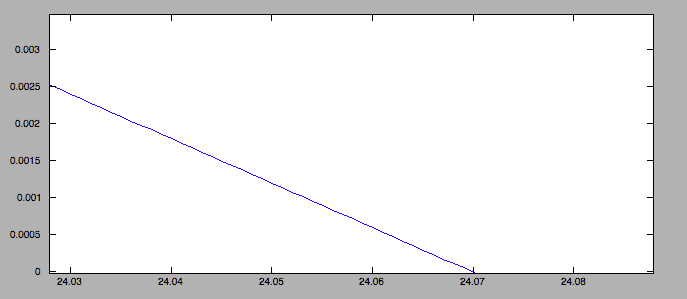# Plotting in TikZ

Gold Member
Hi PF!

I'm plotting a line in TikZ using the following code

Code:
\draw[domain=0:25,samples=100,color=blue,variable=\x,line width=1.5] plot (\x,{0.9924686128641765 - 0.023406305144575135*\x -
0.0006942621866861979*\x^2 - .000001925581045950128*\x^3});

The largest zero is 24.0699 but latex is plotting that zero much larger. I've attached a picture so you can see what I'm referring to. Any ideas as to why this is happening?

#### Attachments

•Screen Shot 2016-05-19 at 11.05.15 AM.png
60.2 KB · Views: 594

jedishrfu
Mentor
Could it have something to do with incremental error?

What if you change the stepping to be more or less steps? and larger or smaller steps?

Gold Member
Could it have something to do with incremental error?

What if you change the stepping to be more or less steps? and larger or smaller steps?

Thanks for replying! I changed the samples to 5, 10, 100 and 1000 and nothing makes this graph converge to it's true zero.

jedishrfu
Mentor
This may be a problem with latex. Perhaps it can't handle the precision of your numbers in doing the calculation.

I tried it in freemat (free matlab clone) and got this pic:which seems to hit the 24.0699 zero.

Gold Member
Yea, you're right, tikZ can only plot to so much precision. First 5 digits after the decimal. I believe there is a way to subvert this though; I'll post if anyone wants the solution?

jedishrfu
Mentor
Post the solution. We live in an asynchronous world where someone 5000 years from now will read your post and will want to know how you subversively tricked TikZ in giving up the answer.

My guess is you multiplied all the constants by some magic number so that they fall into the 5 digit realm and then had TikZ solve for it.

Gold Member
My guess is you multiplied all the constants by some magic number so that they fall into the 5 digit realm and then had TikZ solve for it.

You're guess is spot on! Code is here
Code:
\draw[domain=0:25,samples=100,color=blue,variable=\x,line width=1.5] plot (\x,{0.9924686128641765 - 2.3406305144575135*(\x/100) - 6.942621866861979*(\x/100)^2 - 1.925581045950128*(\x/100)^3});

Thanks for taking the time to consider this!

•jedishrfu